# CURRENT ELECTRICITY

Current electricity is the rate at which an electricity source will make charges to flow or pass a certain point in a conductor or in an electric circuit.

This means that, when electrical devices Electric current is the rate of charge flow past a given point in an electric circuit, measured in Coulombs/second which is named Amperes. In most DC electric circuits, it can be assumed that the resistance to current flow is a constant so that the current in the circuit is related to voltage and resistance by Ohm's law. The standard abbreviations for the units are 1 A = 1C/s.

Current Electricity
Define current electricity
Current electricity is a fundamental quantity and is the amount of charge passing a given point in a circuit divided by the time required for the passage of charges.
Electrical current (I) =quantity of charge (Q)/Time (t)
I =Q/t
Q = I.t
Electric current = rate of flow of charge
= (the number of charge carried per second x charge of a single electron)
From this definition the SI unit of an electric current is I =Columbus(C)/Second (s)
I = c/s = A
This unit is commonly known as an Ampere (A). Other units are milliamperes (mA), kilo amperes (KA) and Microampere (mA).
Their equivalents to the ampere are as follows:
1A = 10-3mA
1A= 10-6mA
1KA = 1000A
So when a steady electric current of 1A is flowing in a circuit a coulomb of charge passes a given point of the circuit per second.
An instrument used to measure electric current is called an Ammeter.
In this chapter we shall study the sustained movement of electric charge called electric current. To maintain a steady flow of electricity charge capable of moving and ways of causing them to move. Secondly, there must be a closed path around which the charge moves. This path is known as electric circuit.
A coulomb
This is the quantity of electricity, which passes a given point in circuit in 1 second when a steady current of 1 ampere flows.
In electric current there are flows of electrons through the conductor. Electrons are negatively charged while protons are positively charged. The motion of the charge through the circuit transfers energy from one point to another. This means that the actual directors of an electric current are opposite to the conventional direction.
Uses of current electricity
Current electricity is mainly used for:
1. Cooking
2. Lighting
3. Communication; and
4. Heating among many other uses
Different Sources of Current Electricity in Everyday Life
Identify different sources of current electricity in everyday life
All sources of electric currents work by converting some kind of energy into electrical energy. The two basic sources are:
1. Batteries e.g. Mobile phone battery, car dry cell batteries and also car alternator.
2. Generator
Batteries convert chemical energy into electrical energy. While generators convert mechanical energy into electrical energy.
Other sources of electric energy include water (hydroelectric power), water currents i.e. ocean waves, solar energy and wind energy.
Hydroelectric power is very reliable except in time of severe drought. This is because electricity is generated from water in dams and waterfalls, which depends on rainwater. Turbines are used to generate electricity form falling water.
Solar cells trap and convert solar energy into electric energy. Space ships and satellite use solar cell to convert sun light into electricity.
COMBINATION OF RESISTORS
Resistors can be connected either in series or parallel depending on the magnitude of effective resistance required. Series connection gives a bigger value of effective resistance and the parallel connection gives small value of effective resistance.
RESISTORS IN SERIES
By connecting resistors in series, when the switch 'S' is closed, the current 'I' which flows through the circuit flows through each resistor.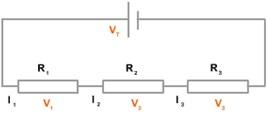Total resistance between points A and B which is commonly referred to as equivalent resistance (Req) will produce a potential difference in the circuit given by ohm’s law as;
V=IReq
The voltage across each resistor in the circuit is given by V1 =IR1 and V2 =IR2
The sum of the voltage drops equal to the potential difference in the circuit (i.e. potential difference between (A and B)
Total voltage = V1 + V2
... VT = V1 + V2
Total voltage = V1 + V2
Since V = IR, V1 = IR1 and V2 = IR2
IRT = IR1 + IR2
IRT =I (R1 + R2 )
RT = R1 + R2

RESISTORS IN PARALLEL
In the figure below I is the current in the main circuit. On the other hand I1 and I2 are current through individual resistors R1 and R2.
The sum of all currents through the resistors which are connected in parallel gives the value of current equal to the main circuit.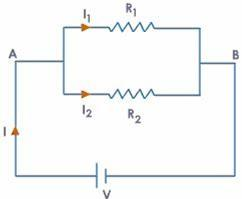.
Therefore, IT = I1 + I2
If RT is the equivalent resistance of the main circuit between A and B, then by Ohm’s law the current is given by;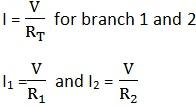From IT = I1 + I2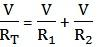On diving both sides by VCross multiplication
1(R1R2) = RT (R1 + R2)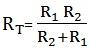For two resistors connected in parallel.
EXAMPLES

1.Given that R1= 4Ω and R2= 6Ω, find the equivalent resistance when the resistors are connected.
1. In parallel
2.   In series

Solution

1.Series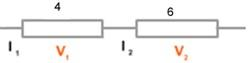RT = R1+R2
RT = 4Ω + 6Ω
RT = 10Ω

2.Parallel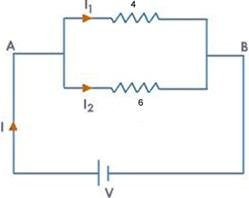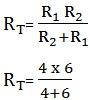= 2.4Ω

2.Two conductors of resistance 4Ω and 5Ω are connected in series across a 60V supply. Find;
1. The total resistance
2. The current in the circuit
3. The potential difference across each resistor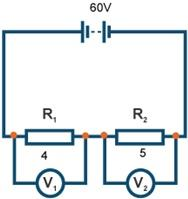RT = R1+R2
= 4Ω + 5Ω
= 9Ω
the total resistance = 9Ω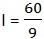I = 6.7A

Potential difference across R1
V1 = IR1
V1 = 6.7 x 4 = 26.8v
Potential difference across R2
V2 = IR2
V2 = 6.7 x 5 = 33.5v
Total current = 26.8 + 33.5 = 60A
3.Consider the circuit shown below. What will be the reading on the Ammeter?
Solution
V = 12V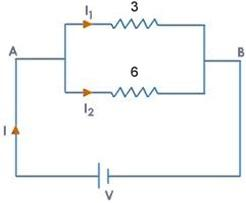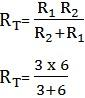RT =2Ω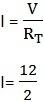I = 6A
EXERCISE

1. In a circuit, the amount of charges passing through a point is 9 coulombs in 4.5 seconds. What is the electric current passing at that point?
Solution
Quantity of charges = 9 coulombs
Time = 4.5 sec
Electric current =?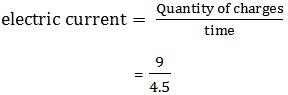Electric current = 2coulombs/sec

2. The two resistances 15Ω and 5Ω are connected in series across 20v supply, find;
1. Total resistance
2. The total current in the circuit
3. The current through each resistor
Solution
Data given
R1 = 15Ω
R2 = 5Ω
Voltage = 20v

The total resistance
RT = R1 + R2
= 15Ω + 5Ω
= 20Ω

The total current in a circuit (I)
From V = IR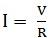But v = 20v, R = 20Ω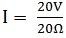I = 1A

The current through each resistor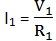But V = 20V, R1 =15Ω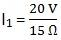= 1.3A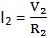but V = 20 V, R = 5Ω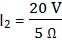I2 = 4A